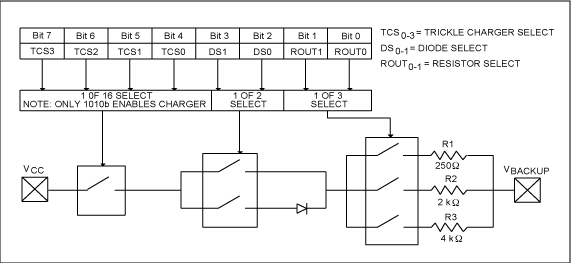# Estimating Super Capacitor Backup Time on Trickle-Charger Real-Time Clocks

### Abstract

The Maxim real-time clock (RTC) family includes a number of parts with integrated trickle-charging circuitry. The trickle charger can be used to charge a secondary battery or capacitor. The battery or capacitor is used to maintain operation of the clock when the supply voltage on VCC is absent. The energy stored in the capacitor will maintain clock operation for a period of time that is determined by several factors. This application note discusses methods used to calculate backup time based on capacitor size.

Also see application note 3816, "Selecting a Backup Source for Real-Time Clocks."

### The Charging Circuit

A typical trickle-charger circuit diagram is shown in Figure 1. A specific four-bit pattern in the upper nibble of the trickle-charger register is used to enable the trickle charger. The lower four bits are used to select a voltage-dropping diode and current-limiting resistor. In the diagram below, either one diode or no diode can be inserted into the charging path, and the resistor values that can be selected are 250Ω, 2kΩ, or 4kΩ. Some devices provide different diode and resistor configurations (check the device data sheet for details). The capacitor is connected from VBACKUP to ground (Figure 2).The user determines the diode and resistor selection according to the maximum current required for capacitor charging. Contact the manufacturer of the capacitor or check the capacitor data sheet for charging-current limits.

### Charging-Current Calculations

The maximum charging current can be calculated as follows: assume that a system power supply of 3.3V is applied to VCC, and that the trickle charger has been enabled with no diode and a 2kΩ resistor. The maximum current, when the capacitor voltage is zero, would be calculated as:

IMAX = (VCC - diode drop)/R2
= (3.3V - 0V)/R2
≈ (3.3V - 0V)/2kΩ
≈ 1.65mA

As the voltage on VBACKUP increases, the charging current decreases.

### Calculating Backup Time

Now we need to determine how large the capacitor needs to be. Given the desired backup time, we need to know several other parameters: the starting and ending voltage on the capacitor, the current draw from the capacitor, and the capacitor size.

If we assume that the RTC draws a constant current while running from VBACKUP, then calculating the worst-case backup time in hours would use the formula:

[C(VBACKUPSTART - VBACKUPMIN)/IBACKUPMAX]/3600

Where:

C is the capacitor value in farads

VBACKUPSTART is the initial voltage in volts (the voltage applied to VCC, less the voltage drop from the diodes, if any, used in the charging circuit)

VBACKUPMIN is the ending voltage in volts (the minimum oscillator operating voltage)

IBACKUPMAX is the maximum data sheet VBACKUP current in amps

Given that C = 0.2F, VBACKUPSTART = 3.3V, VBACKUPMIN = 1.3V, and IBACKUPMAX = 1000nA, then:

Hours = [0.2(3.3 - 1.3)/(1e - 6)]/3600 = [0.2(2.0)/(1e - 6)]/3600 = 111.1

If we want to know what the typical backup time should be, we would substitute the IBACKUP typical value (IBACKUPTYP)for IBACKUP maximum (IBACKUPMAX).

Therefore, if VBACKUPTYP is 3.3V (typ) and IBACKUPTYP is 600nA (typ), then:

Hours = [0.2(3.3 - 1.3)/(600e - 9)]/3600 = [0.2(2.0)(600e - 9)]/3600 = 185.2

These calculations assume that IBACKUP is constant, regardless of the voltage on VBACKUP. The oscillator on Maxim RTCs tends to act more like a resistor, so that backup current tends to decrease with the backup voltage. It should, therefore, be possible to calculate a more realistic backup time.

From basic electronics, the formula to determine the voltage across a capacitor at any given time (for the discharge circuit in Figure 3) is:

V(t) = E(e-τ/RC)Where:
τ is the time in seconds
E is the initial voltage in volts
V is the ending voltage in volts
R is the resistive load in ohms
C is the capacitor value in farads

Rearranging the equation to solve for t, we get:

-ln(V/E)(RC) = t

From the RTC data sheet, we can get the minimum oscillator operating voltage as well as the maximum VBACKUP current (IBACKUPMAX). To estimate the load resistance, R, we divide the data sheet VBACKUPMAX by IBACKUPMAX (because the worse-case current occurs at the maximum input voltage). For this example, VBACKUPMAX is 3.7V and IBACKUPMAX is 1000nA, or 3.7/1e-6 or 3,700,000Ω. Assuming that the capacitor value is 0.2F and has been charged to 3.3V, that the IBACKUPMAX is 1000nA, and that the minimum oscillator operating voltage is 1.3V, the backup time would be calculated as:

-ln(VBACKUPMIN/VBACKUPMAX)[(VBACKUPMAX/IBACKUPMAX) × C] =
-ln(1.3/3.3)(3,700,000 × 0.2) =
689,353s (191.5hrs)

By changing the value of C, the estimated operating time while running from the backup capacitor can be determined.

These calculations can be done using the on-line calculator. This Supercapacitor Calculator implements the three equations shown above.